MORE IN Signals & Systems
Total marks: --
Total time: --
INSTRUCTIONS
(1) Assume appropriate data and state your reasons
(2) Marks are given to the right of every question
(3) Draw neat diagrams wherever necessary

1 (a) Determine the even and odd part of the signal x(t) shown in Fig. Q1(a).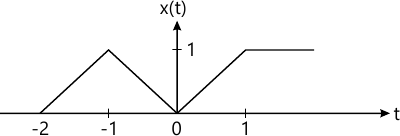6 M
1 (b) The signal x1(t) and x2(t) are shown in Fig 1(b). Sketch the following signals:
i) x1(t) + x2(t)
ii) x1(t).x2(t)
iii) x1(1/2)
iv) x2(2t)
v) x2(t) x1(t)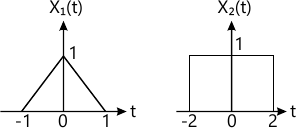8 M
1 (c) Check whether each of the following signals is periodic or not. If periodic determine its fundamental period: $i) \ x(n)= \cos (2n) \\ ii) \ x(n)= (-1)^n \\ iii) \ x(n)=\cos \left ( \dfrac {\pi}{8} n^2 \right )$
6 M

2 (a) Perform the convolution of the following signals shown in Fig. Q2(a) and also sketch the o/p signal y(t).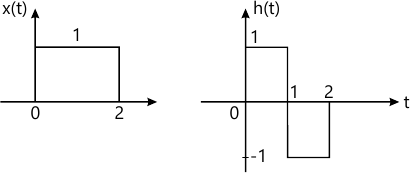8 M
2 (b) Compare the convolution sum of
x(n)-?n[u(n)-u(n-8)], |?|<1 and h(n)=u(n)-u(n-5).
8 M
2 (c) Compute the convolution of two sequences
x1(n)={1, 2, 3} and x2(n)={1, 2, 3, 4}
4 M

3 (a) Check the followings are stable, causal and memoryless;
i) h(t)=e-tu(t+100)
ii) h(t)=e-4|t|
iii) h(n)=2u(n)-2u(n-2)
iv) h(n)=?(n)+sin(n?).
8 M
3 (b) Find the total response of the system given by $\dfrac {d^2y(t)}{dt^2}+3 \dfrac {dy (t)}{dt}+2y(t)=2x(t) \ with \ y(0)=-1, \dfrac {\frac {dy(t)}{dt}}{t=0}=1 \ and \ input \ x(t)-\cos t\ u(r)$
7 M
3 (c) Find the difference equation corresponding to the block diagram shown in Fig Q3(c).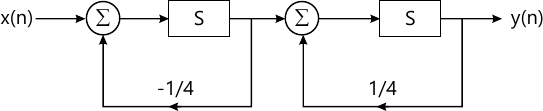5 M

4 (a) $\displaystyle If \ x(n)\overset{DTFS}{\leftrightarrow} X(k) \ and \ y(n) \overset{DTFS}{\leftrightarrow} Y(k), \ then \ prove \ that \ x(n).y(n) \overset{DTFS}{\leftrightarrow} X(k)\circledast Y(k).$
7 M
4 (b) Obtain the DTFS coefficients of $x(n)\cos \left ( \dfrac {6\pi}{13}n + \dfrac {\pi}{6} \right ).$ Draw the magnitude and phase spectrum.
6 M
4 (c) Determine the time domain corresponding to the following spectra shown in Fig Q4(c)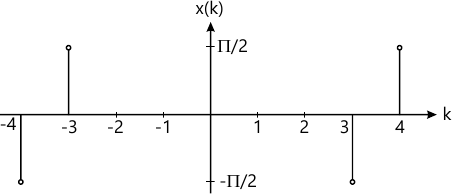7 M

5 (a) Let F{x1(t)}=x1(j?) and F{x2(t)}=x2(j?) then prove that $Let \ F\{x_1(t)\}=x_1(j\Omega) \ and \ F\{ x_2 (t)\}=x_2(j\Omega) \\ then \ prove \ that \\ F\{ x_1(t). x_2(t)\}= \dfrac {1}{2\pi} \int^{\infty}_{\lambda=-\infty} x_1 (j\lambda)x_2 (j\Omega-\lambda)d\lambda$
7 M
5 (b) Find the Fourier transform of the signal x(t) shown in Fig Q5(b)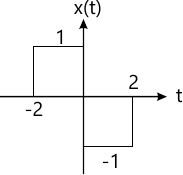6 M
5 (c) Find the inverse Fourier transform of $X(jw)= \dfrac {jw}{(2+jw)^2}$ using properties.
7 M

6 (a) Draw the frequency response of the system described by the impulse response h(t)-?(t)-2e-2t u(t).
7 M
6 (b) Find the Fourier transform of the periodic impulse train $\delta_{To}(t)= \sum^{\infty}_{k=-\infty}\delta (t-kTo)$ and draw the spectrum.
8 M
6 (c) A signal x(t)=cos(10?t)+3cos(20?t) is ideally sampled with sampling period Ts. Find the Nyquist rate.
5 M

7 (a) Determine Z-transform of the following DTS and also find the ROC: $i) \ x(n)=0.8^n u(n-1) \\ ii) \ x(n)=-u(-n+1)+ \left ( \dfrac {1}{2} \right )^n u(n)$
8 M
7 (b) $If \ x(n) \overset{z}{\leftrightarrow}X(z), \ with \ ROC=R \ then \ prove \ that \ n.x(n) \overset{z}{\leftrightarrow}-z\dfrac {X(z)}{dz} \ with \ ROC-R.$
6 M
7 (c) Determine the inverse Z-transform of the function $X(z)= \dfrac {3z^2+2z+1}{z^2+3z+2}$
6 M

8 (a) Determine the impulse response of the sequence describe by y(n)-2y(n-1)+y(n-2)=x(n)+3x(n-3).
8 M
8 (b) Solve the following difference equation using unilateral z-transform. $y(n) - \dfrac {3}{2} y(n-1)+ \dfrac {1}{2}y(n-2)=x(n), \ for \ n\ge 0, \ with \ initial \ conditions \ y(-1)=4, y(-2)=10, \ and \ i/p \ x(n)= \left ( \dfrac {1}{4} \right )^n u(n)$
8 M
8 (c) Define stability and causality with respect to z-transform.
4 M

More question papers from Signals & Systems